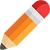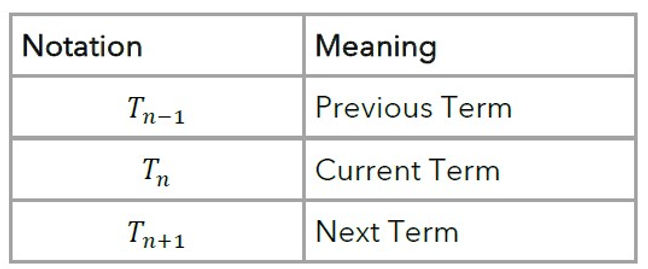top of page# Learning Objectives###### ​###### What are sequences?Sequences are sets of items belonging in a specific order, according to a certain rule.

Each member of a sequence is referred to as a term.

An example of a simple sequence is 5, 10, 15, 20, 25.

Consider the terms of the above sequence. The first term is 5. We write this as T₁=5. The second term is 10, which is written as T₂=10. And, since the third term is 15, T₃=15.###### Sequence notationWhen dealing with sequences, we use a special notation. The above table summarises this.###### Types of sequencesWe will be required to work with two main types of sequences: arithmetic sequences (also known as APs) and geometric sequences (or GPs).

An Arithmetic Progression (AP) is some sequence of numbers in which each term is obtained from the previous term by the addition of some constant number.

A Geometric Progression (GP) is one where the next term in the sequence is obtained from multiplying the previous term by a constant number.###### Arithmetic sequences / progressions (APs)Sequences of numbers in which each term is obtained from the previous term by the addition of some constant number are said to be arithmetic sequences or APs.

Thus, all arithmetic sequences are of the form:

𝒂, 𝑎+𝒅, 𝑎+2𝑑, 𝑎+3𝑑, 𝑎+4𝑑, 𝑎+5𝑑, 𝑎+6𝑑.

In this general form, we have:

• A first term of 𝒂

• A common difference of 𝒅.

Therefore, Tₙ₊₁ = Tₙ + d.###### Geometric sequences / progressions (GPs)All GPs are of the form 𝒂, 𝑎𝒓², 𝑎𝑟³, 𝑎𝑟⁴, 𝑎𝑟⁵, 𝑎𝑟⁶, 𝑎𝑟⁷.

In this general form, we have:

• A first term of a; and

• A common ratio of r.

Using recursive notation, we write this as Tₙ₊₁ = 𝑟×Tₙ with T₁=𝑎.###### ​###### ​###### ​bottom of page